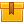# Positive and Negative Numbers, Powers, and Roots (A4305)

## Prerequisites

This lesson is designed to build on the skills presented in the fourth lesson of this program, Percent, Ratio, and Proportion.

## Description

This lesson introduces the concepts of positive and negative numbers, powers and roots. Participants learn how to use positive and negative numbers in addition, subtraction, and multiplication. Additionally, the lesson explains formulas and how to use them. The concepts presented in this lesson are designed to prepare participants for algebra.

## Objectives

Describe the concept of positive and negative numbers. Recognize positive and negative values and their opposites. Add, subtract, multiply and divide positive and negative numbers using pencil and paper. Use the plus/minus key to enter negative values on the calculator. Add, subtract, multiply and divide positive and negative values using a calculator. Use positive and negative numbers to describe deviation from a target value. Recognize a power. Describe the concept of a squared number. Square numbers by multiplication using pencil and paper. Square numbers by multiplication and by using the square key on a calculator. Describe the concept of a square root. Recognize perfect squares and their square roots. Use the square root key on a calculator to find square roots. Estimate answers to problems that involve positive and negative numbers, squares and square roots. Use formulas that involve squares and square roots. Solve problems involving positive and negative numbers, powers and roots.

## ContentThis lesson introduces the concepts of positive and negative numbers, powers and roots. Participants learn how to use positive and negative numbers in addition, subtraction, and multiplication. Additionally, the lesson explains formulas and how to use them. The concepts presented in this lesson are designed to prepare participants for algebra.

•Positive and Negative Numbers, Powers, and Roots (A4305)

0
0 Reviews